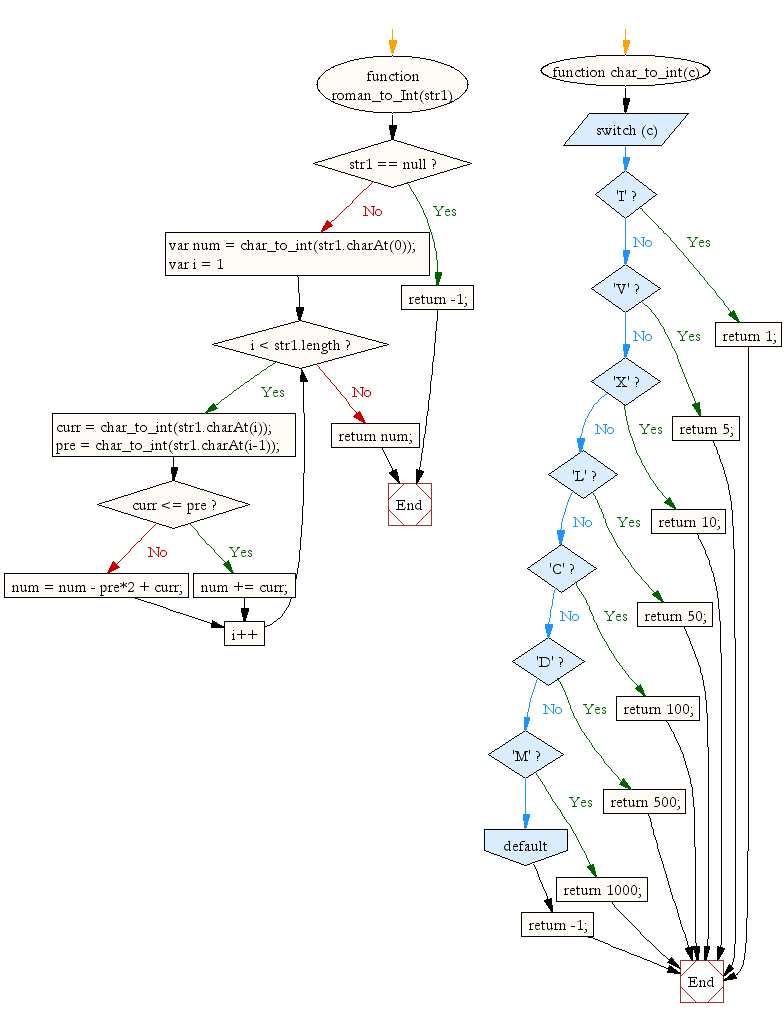# JavaScript: Convert Roman Numeral to Integer

## JavaScript Math: Exercise-22 with Solution

Write a JavaScript function that Convert Roman Numeral to Integer.

Sample Solution:-

HTML Code:

``````<!DOCTYPE html>
<html>
<meta charset="utf-8">
<title>Write a JavaScript function that Convert Roman Numeral to Integer</title>
<body>

</body>
</html>
```
```

JavaScript Code:

``````function roman_to_Int(str1) {
if(str1 == null) return -1;
var num = char_to_int(str1.charAt(0));
var pre, curr;

for(var i = 1; i < str1.length; i++){
curr = char_to_int(str1.charAt(i));
pre = char_to_int(str1.charAt(i-1));
if(curr <= pre){
num += curr;
} else {
num = num - pre*2 + curr;
}
}

return num;
}

function char_to_int(c){
switch (c){
case 'I': return 1;
case 'V': return 5;
case 'X': return 10;
case 'L': return 50;
case 'C': return 100;
case 'D': return 500;
case 'M': return 1000;
default: return -1;
}
}
console.log(roman_to_Int('XXVI'));
console.log(roman_to_Int('CI'));
```
```

Sample Output:

```26
101
```

Pictorial Presentation:Flowchart:Live Demo:

See the Pen javascript-math-exercise-22 by w3resource (@w3resource) on CodePen.

Improve this sample solution and post your code through Disqus

What is the difficulty level of this exercise?

﻿

## JavaScript: Tips of the Day

Errors

```const name = 'Owen';

console.log(name());
```

The variable name holds the value of a string, which is not a function, thus cannot invoke.
TypeErrors get thrown when a value is not of the expected type. JavaScript expected name to be a function since we're trying to invoke it. It was a string however, so a TypeError gets thrown: name is not a function!
SyntaxErrors get thrown when you've written something that isn't valid JavaScript, for example when you've written the word return as retrun. ReferenceErrors get thrown when JavaScript isn't able to find a reference to a value that you're trying to access.

Ref: https://bit.ly/3jFRBje